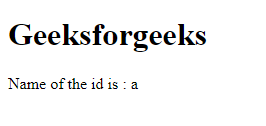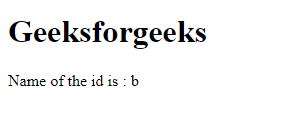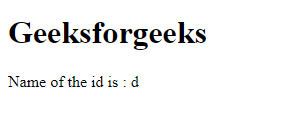Related Articles

# How to print object by id in an array of objects in JavaScript?

• Last Updated : 22 Feb, 2021

We have an array of objects and in every object, there is a key named id and whose value is a number.

Example:

```const arr = [
{
'id' : 12,
'name' : 'Ram'
},
{
'id' : 32,
'name' : 'Shyam'
}
]```

We want to find the value of the name property whose id number is n.

Example :

```Input : 12
Output : Ram

Input : 32
Output : Shyam```

There are many approaches to solve this problem which are following

Using Array.filter( ) Array.filter( ) method is used for creating a new array from an existing array after applying some conditions.

## HTML

 ```<``html``>``    ``<``body``>``        ``<``h1``>Geeksforgeeks`` ` `        ``<``p``>Name of the id is : ``          ``<``span` `id``=``"geeks"``>``        ```` ` `        ``<``script``>``           ` `            ``// This is our array of Objects``            ``var data = [``                ``{ id: 1, name: "a" },``                ``{ id: 2, name: "b" },``                ``{ id: 3, name: "c" },``                ``{ id: 4, name: "d" },``                ``{ id: 5, name: "e" },``                ``{ id: 6, name: "f" },``            ``];`` ` `            ``let idYouWant = 1;``            ``let propertyYouWant = "name";`` ` `            ``// Using Array.filter( ) method``            ``// we are iterating through each``            ``// items in the array and``            ``// checking which item's``            ``// id value is equal to the id we want`` ` `            ``let res = data.filter((item) => {``                ``return item.id == idYouWant;``            ``});`` ` `            ``// After using filter method we got an array``            ``// of object. Now take its first element and``            ``// use its 'propertyYouWant' key``            ``let exactRes = res[propertyYouWant];`` ` `            ``// Printing the property we want``            ``document.getElementById("geeks").innerText = exactRes;``        ````    `````

Output:Using Array.find( ): Using Array.find( ) first we are searching in which object the given id exists, then we extract the name property from that object.

## HTML

 ```<``html``>``    ``<``body``>``        ``<``h1``>Geeksforgeeks`` ` `        ``<``p``>Name of the id is : ``          ``<``span` `id``=``"geeks"``>``        ```` ` `        ``<``script``>``           ` `            ``// This is our array of Objects``            ``var data = [``                ``{ id: 1, name: "a" },``                ``{ id: 2, name: "b" },``                ``{ id: 3, name: "c" },``                ``{ id: 4, name: "d" },``                ``{ id: 5, name: "e" },``                ``{ id: 6, name: "f" },``            ``];`` ` `            ``let idYouWant = 2;``            ``let propertyYouWant = "name";`` ` `            ``// Using Array.find( ) we are searching``            ``// in which object our searching id present`` ` `            ``let res = data.find((item) => {``                ``return item.id == idYouWant;``            ``});`` ` `            ``// Now print the property which you want from``            ``// the object res console.log(res[propertyYouWant])``            ``document.getElementById("geeks").innerText = ``                    ``res[propertyYouWant];``        ````    `````

Output:Using for loop Using for loop first we are iterating the array and searching in which object the given id present and after that, we are printing the property we wanted.

## HTML

 ```<``html``>``    ``<``body``>``        ``<``h1``>Geeksforgeeks`` ` `        ``<``p``>Name of the id is :``          ``<``span` `id``=``"geeks"``>``        ```` ` `        ``<``script``>``            ``// This is our array of objects``            ``var data = [``                ``{ id: 1, name: "a" },``                ``{ id: 2, name: "b" },``                ``{ id: 3, name: "c" },``                ``{ id: 4, name: "d" },``                ``{ id: 5, name: "e" },``                ``{ id: 6, name: "f" },``            ``];`` ` `            ``let idYouWant = 4;``            ``let propertyYouWant = "name";`` ` `            ``// Iterating over the array using for loop and``            ``// searching in which object the id present``            ``// After getting the object we print the``            ``// property we wanted from the object`` ` `            ``for (var i = 0; i < ``data.length``; i++) {``                ``if (data[i].id == idYouWant) {``                    ``// console.log(data[i][propertyYouWant])``                    ``document.getElementById("geeks")``.innerText` `= ``                            ``data``[i][propertyYouWant];``                ``}``            ``}``        ````    `````

Output :Hey geek! The constant emerging technologies in the world of web development always keeps the excitement for this subject through the roof. But before you tackle the big projects, we suggest you start by learning the basics. Kickstart your web development journey by learning JS concepts with our JavaScript Course. Now at it’s lowest price ever!

My Personal Notes arrow_drop_up